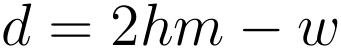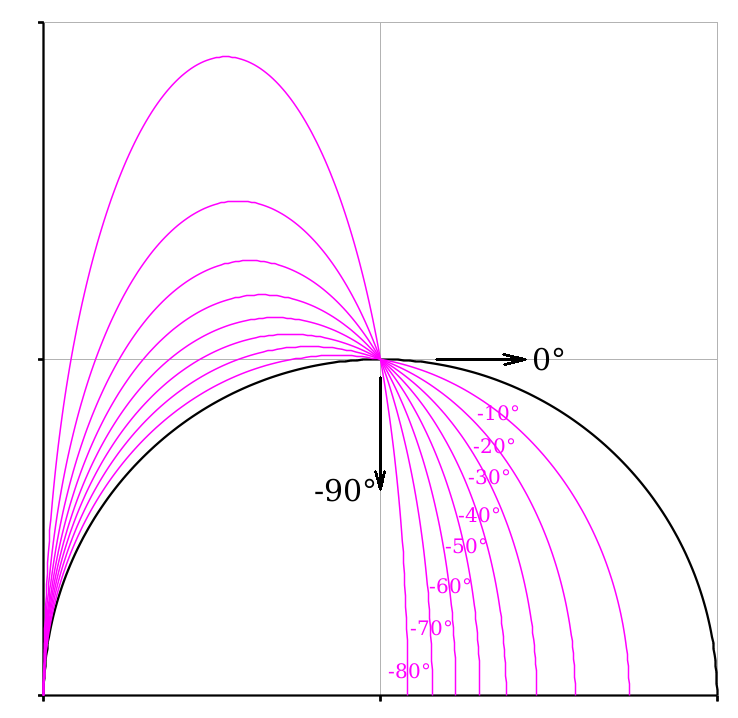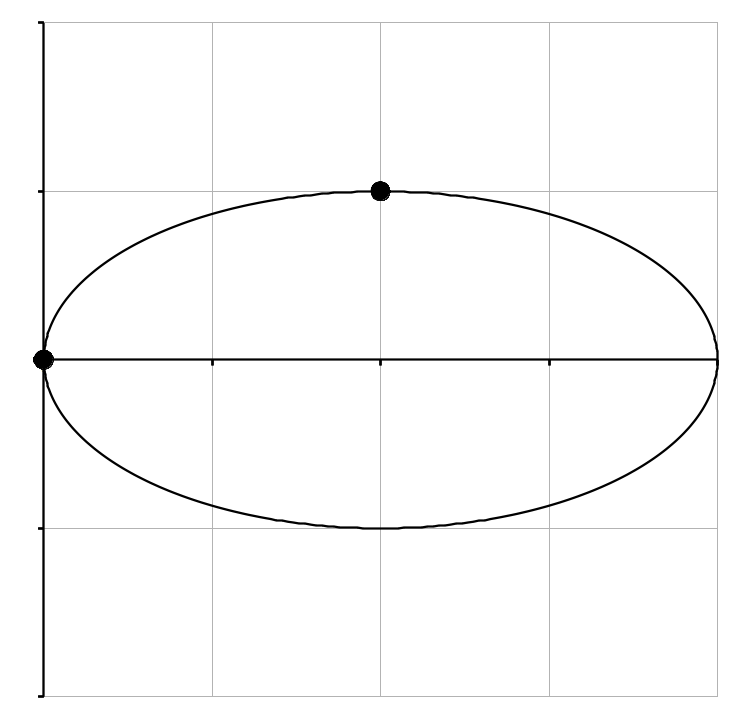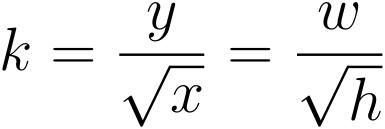Claus Johansen, Sønderborg, Danmark, 2019-07-16 -> 2020-01-14

# Construct a conic by two points and two tangents

Indhold:

## 0) Preface

The curves created by conics (circle, ellipse, parabola and hyperbola) have several good properties, when modelling geometri.

• They are very "stif", in the sense, that they don't overshoot, at sharpe corners.
• They have a well-defined curvature along the full curve.

It can be practical to define, which conic connets two points, when a specific angle is demanded in these two points.

There is a continous transition between the different conics: circle, ellipse, parabola and hyperbola.

When it's stated here, that you can define a conic by two two points and two tangents, it's a mild exaggeration - the one point must be a top-point, so that the centre-line thereby is known.

## 1) The task

An example is shown below. The one point is placed in the centre of the coordinate system and has thereby the coordinates (0, 0) - this point is a top-point and the x-axis is symmetri-axis for the conics that are determined here. The other point is placed in the coordinates (h, w):

Where:
h ~ the height of the points position (=x coordinate).
w ~ the width of the points position, or the half width of the conic at this place (=y coordinate).Fig. 1.1: The task.

## 2) The solution, ellipse and hyperbola separately

The formulas were first deduced for each type of conic separatly, and lead to the following solutions:

No Ellipse Hyperbola property
1= do Slope of the tangent in the point (h, w)
2= do Slope of the radius to the point (h, w)
3a (semi axis along x-axis)
4Dim.less b (semi axis parallel to y-axis)
5= do b (semi axis parallel to y-axis)

At a closer inspection, it's easy to see, that ther's only minor differences between the equations in column 2 and 3: "Ellipse" and "Hyperbola" - it's actually only change of sign, that keeps them apart. It's therefore obvious to combine them to one set of equations - that will be done in the next section.

## 3) The solution, ellipse and hyperbola collectively

The slope is evaluated first:(3.1)

Then the property "d" is evaluated - it determines the type of conic (it can be recognised as part of the expressions in row 2, in the table in the previous section, where "τ" is evaluated):(3.2)
• d < 0 ⇒ ellipse
• d = 0 ⇒ parabola
• d > 0 ⇒ hyperbola

Thereafter τ:(3.3)

The semi axis along the X-axis:(3.4)

The dimensionless semi axis parallel to the Y-axis:(3.5)

Thereby the semi axis parallel to the Y-axis becomes:(3.6)

Some examples of possible solutions are shown in the figure below (there are no solutions in the gray coloured areas):Fig. 3.1: Examples of solutions.

There are a couple reservation in this solution:

• h ≠ 0, because h = 0 don't give any meaning.
• w ≠ 0, because w = 0 don't give any meaning.
• m ≠ 0, because m = 0 gives an ellipse, that must be determined in another way.
• d ≠ 0, because d = 0 gives a parabola, that must be determined in another way.

These reservation will be treated i § 5.

## 4) The solution divided into regions

The regions with uniform solutions are examined systematically below , where θ take values from -90° to +90°.

## 4.a) -90° < θ < 0°Fig. 4.1: -90° < θ < 0°.

NB: The value for θ of 0° is only valid in this example.

By comparing the semi-axes of the ellipse, a and b, you will find:

• a < b   ⇒   "standing" ellipsee as shown above.
• a = b   ⇒   circle.
• a > b   ⇒   "lying" ellipsee as shown below.

## 4.b) 0° < θ < 26.565°Fig. 4.2: 0° < θ < 26.565°.

NB: The value for θ of 26.565° is only valid in this example, the criterion is:
d < 0 ⇒ ellipse

It will still be relevant, to make a check of the shape of the ellipse:

• a < b   ⇒   "standing" ellipses.
• a = b   ⇒   circle.
• a > b   ⇒   "lying" ellipses as shown above.

## 4.c) 26.565° < θ < 45°Fig. 4.3: 26.565° < θ < 45°.

NB: The value for θ of 26.565° is only valid in this example, the criterion is:
d > 0 ⇒ hyperbel

NB: The value for θ of 45° is also only valid in this example, the criterion is, that θ is smaller than the angle of the line that connects the two points:
θ < atan(w/h).

## 4.d) 45° ≤ θ ≤ 90°

There shouldn't be any solutions in this interval, but the equations don't give up with a negative square root or similar. The solutions that appears are hyperbolas, but they don't fulfill the criterion to pass through the point (h, w):Fig. 4.4: 45° ≤ θ ≤ 90°

NB: The value for θ of 45° is also only valid in this example, the criterion is, that θ is smaller than the angle of the line that connects the two points:
θ < atan(w/h).

## 5) The solution at limit values

There was a couple of reservations to the general solution, mentioned in § 3.

Formally you could argue, that with the right angle, there could be solutions to the problem in the form of straight lines, if either h = 0 or w = 0. And further you could argue, that a straight line just is a degenerated conic - this is mostly curiosities...

## 5.a) m = 0

At m = 0 there'll be divided by 0 i eq. 3.3 and 3.4, and the set of equations can't be used. The solution is however very simple.

For m = 0 is the answer simply an ellipse, with the two semi-axis h and w:(5.a.1)(5.a.2)

With the chosen set of numbers (h, w) = (1, 1) the ellipse becomes a circle when m = 0 and thereby θ = 0. This is however a pure coincidence, the solutions for m = 0 are shown below for the set of numbers (h, w) = (1, 2) and (h, w) = (2, 1):Fig. 5.a.1: m = 0 & (h, w) = (1, 2). Fig. 5.a.2: m = 0 & (h, w) = (2, 1).

## 5.b) d = 0

When d = 0 leads to τ = 0 in eq. 3.3 and ther will threby by divided by 0 in eq. 3.4, and the set of equations can't be used. The solution is however very simple.

For d = 0 is the answer simply a parabola.

The equation for a lying parabola can be written as:(5.b.1)

When it's known, that the curve must pass through the point (h, w), it's easy to evaluate the constant c:(5.b.2)

You can also choose an equation, that more directly gives y as function of x:(5.b.3)

When it's still known, that the curve must pass through the point (h, w), it's easy to evaluate the constant k:(5.b.4)Fig. 5.b: The parabola

## 5.c) a = b

There's per se nothing special by the case a = b - it's just an ellipse with to equal axes, in other words a circle. Here it can be seen as a limit-case in the way, thet it separates the ellipses that are standing, from the lying ones.Fig. 5.c.1: Cirkle for (h, w) = (1, 2). Fig. 5.c.2: Cirkle for (h, w) = (2, 1).

## 6) Solutions in the interval 90° -> 270°

The solutions in the interval 90° -> 270° repeats simply the solutions that were found in the interval -90° -> 90°. As it's illustrated below, it doesn't change the analysed conic, if the tangents are rotated 180°:Fig. 6.1: Tangents -90° & 0°. Fig. 6.2: Tangents +90° & 180°.